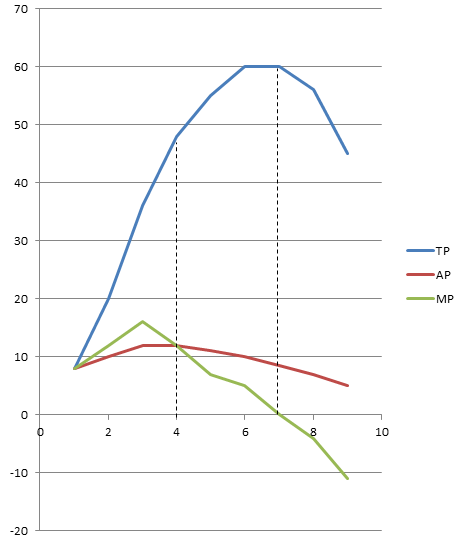# Law of variable production

The law of variable production explains about the short run production phenomenon. In the short run if we want to change output we can change only variable factor but not the fixed factor.

This law states that, if we use more and more units of variable i.e. labor with given fixed factor i.e. capital then in initial phase total production increases at increasing rate to some extent and increases at decreasing rate then reaches to maximum and remains constant after that it start to decline at increasing rate.

This law is based on following assumptions:

Production technology remains constant
Labor is single variable factor which is homogeneous
There must be the possibility of changing the proportion of factors of production

We can explain this concept with the help of following table:

 Fixed factor (i.e. Capital) Variable factor (i.e. Labor) TP AP = TP/L MP = ∆TP/∆L Stage of Production 2 1 8 8 8 1st stage 2 2 20 10 12 2 3 36 12 16 2 4 48 12 12 2 5 55 11 7 2nd stage 2 6 60 10 5 2 7 60 8.57 0 2 8 56 7 -4 3rd stage 2 9 45 5 -11

The above table shows when the more and more labor are used then TP increases at increasing rate to some extent then increases at decreasing rate. AP gradually increases and reaches to maximum. MP sharply increases and reaches to the maximum and starts to decline> In second stage TP increases at decreasing rate, reaches to the maximum. AP gradually decline, MP tends to zero. In the third stage, TP starts to decline, AP gradually declines and MP negatively decline. The same concept can be explained by the given figure.On the above figure we can observe three stage of production which is given below:

1. 1st Stage:
First stage covers 0 to L1. In this stage TP increases at increasing rate to point A and increases at decreasing rate. AP gradually increases and MP gradually increases, reaches to maximum and starts to fall. At the ending point of 1st stage AP =MP.

In the 1st stage increasing return to scale crop up due to the increase in efficiency of fixed factor and variable factor.

2. 2nd Stage:
Second stage covers L1 to L2. In this stage TP increases at decreasing rate and reaches to the maximum. AP gradually declines; MP also declines and reaches to zero. In the second stage diminishing return to scale crop up due to the imperfect substitution of factors of production and scarcity of resources.

3. 3rd Stage:
Third stage starts from L2. In this stage TP increases at increasing rate, AP gradually decreases, MP negatively declines. In the third stage negative return to scale crop up.